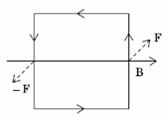Question

A square current carrying loop is suspended in a uniform magnetic field acting in the plane of the loop. If the force on one arm of the loop is F, the net force on the remaining three arms of the loop is:

A
3FB
FC
3FD
FSolution

The correct option is B $$-F$$A square current carrying loop is suspended in a uniform magnetic field acting in the plane of the loop. If the force on one arm of the loop is F, the net force on the remaining three arms of the loop is Force on remaining arms = − FPhysics

Suggest Corrections0Similar questions
View MorePeople also searched for
View More